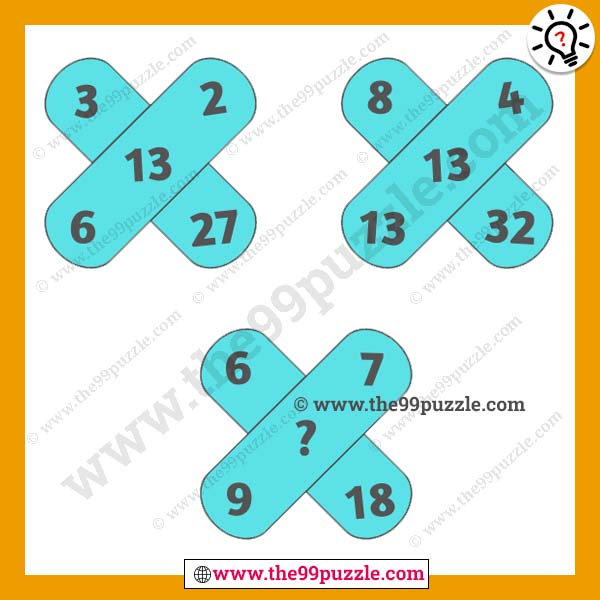# Brainteaser math puzzle tricks with answer – Puzz288

These brainteaser math puzzle tricks will stretch your brain. If you want good puzzlers, you have to practice all these puzzles. Most puzzlers are a very genius and solve this tricky puzzle very quickly. Here you see three simple problems with the same math logic. You have to crack the math logic and replace the question mark. Can you challenge your brain by solving the same logic in various math puzzles? If you can practice these puzzles every day, you can solve any exam very quickly.###### Explanation:

1st Figure (Top Left) = (27÷3)+(6-2) = 13

2nd Figure (Top Right) = (32÷8)+(13-4) = 13

3rd Figure (Bottom) = (18÷6)+(9-7) = 5